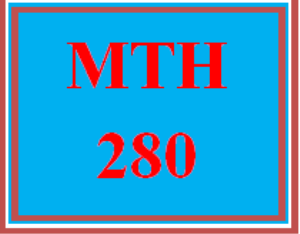# MTH 280 Wk 7 – Final Exam

PLDZ-13200 Free In Stock
\$ 0.00 USD
Description

***************************************************************

~~~~~~~~~~~~~~~~~~~~~~~~~~~~~~~~~~~~~~~~~~~~~~~~~

https://uopcourse.com/

***************************************************************

# MTH 280 Wk 7 – Final Exam

Question 1

A car starts from a point at 2:00 p.m. and travels north at 40 mph. Another car starts from the same point at 3:00 p.m. and travels west at 50 mph. After the second car has traveled 1 h, at what rate is the distance between the two cars changing?

Question 2

A spherical ball is measured to have a radius of 6 space c m with a possible measurement error of plus-or-minus 0.1 rm c m. Use the differentials to estimate the percentage error in computing the volume of the ball.

1%

3%

5%

10%

Question 3

A company determines a cost function of c equals 6 x squared minus 180 x plus 2000, where c is the cost (in dollars) of producing x number of items. How many items should the company manufacture to minimize the cost?

12

15

24

30

Question 4

The position of an object is given by the equation s left parenthesis t right parenthesis equals 2 x squared plus x minus 6. Find the time t at which the instantaneous velocity of the object equals the average velocity in the interval open square brackets 0 comma 3 close square brackets.

t equals 1.5 semicolon space s

t equals 3 semicolon space s

t equals 2 semicolon space s

t equals 0 semicolon space s

Question 5

Find the locations of local minimum and maximum of x to the power of 9 minus 4 x to the power of 8 using the second derivative test.

Local minimum at x equals 0, local maximum at x equals 32 over 9

Local minimum at x equals 32 over 9, no local maximum

Local minimum at x equals 32 over 9, local maximum at x equals 0

Local minimum at x equals 0, no local maximum

Question 6

In a shop, the revenue and the cost of a product are determined by R left parenthesis x right parenthesis equals 22 x and C left parenthesis x right parenthesis equals 2 x squared plus 2 x plus 1, respectively. If x represents the number of products, how many products should the shop sell to maximize the profit?

11

5

6

10

Question 7

Evaluate limit as x rightwards arrow 0 of fraction numerator x squared over denominator e to the power of x minus x minus 1 end fractionby applying L'Hôpital's rule.

Recent Reviews Write a Review
0 0 0 0 reviews